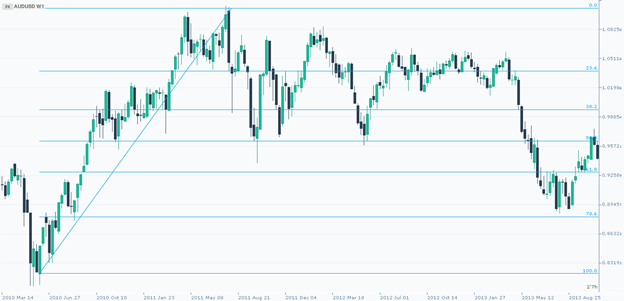Les CFD sont des instruments complexes et présentent un risque élevé de perte rapide d'argent en raison de l'effet de levier. 63% des comptes des investisseurs particuliers perdent de l'argent lorsqu'ils négocient des CFD avec ce fournisseur. Vous devez vous demander si vous comprenez le fonctionnement des CFD et si vous pouvez vous permettre de prendre le risque élevé de perdre votre argent.

Régulateur :

×
###### CySEC

La société Skilling Ltd est réglementée par la Commission de surveillance financière de Chypre (CySEC, Cyprus Securities and Exchange Commission) sous la licence CIF n° 357/18.

###### FSA

La société Skilling (Seychelles) Ltd est autorisée et réglementée par la FSA (Financial Services Authority) sous la licence n° SD042.

# What are Fibonacci Retracements?

Fibonacci Retracements (often called Fibonacci's) are a very popular charting tool amongst technical traders. They take their name from the 13th century mathematician Leonardo Fibonacci. He was very famous in his time (you may have heard of him in other fields), and indeed his ideas were originally not intended for trading at all, given that trading the markets didn’t exist in their modern form during his lifetime.

The Fibonacci numbers can be found in other areas of life. Nature, Art, Architecture.Fibonacci Retracements became more popular over the last few decades in trading because of the mathematical relationships of the sequence, which are expressed as ratios. No one definitively knows why Fibo levels work...although certainly many traders do whilst using them.

Fibonacci sequences are a series of numbers where each number is calculated by adding the two previous numbers. Here is the Fibonacci sequence of numbers that he identified:

0, 1, 1, 2, 3, 5, 8, 13, 21, 34, 55, 89, 144, 233, 377, 610, etc.

In terms of trading, the most important Fibonacci Retracement levels: 23.6% 38.2% , 61.8% , 161.8% which are based on the following calculations:

• The 38.2% ratio is found by dividing one number in the series by the number that is found two places after. For example: 55/144 = 0.3819.
• 61.8% - also referred to as "the golden ratio" is found by dividing one number in the series by the number that follows it. For example: 8/13 = 0.6153, and 55/89 = 0.6179.
• The 23.6% ratio is found by dividing one number in the series by the number that is three places to the right. For example: 8/34 = 0.2352

If you apply other Fibo numbers to these formulas you will notice that some results differ slightly from the above mentioned percentages. This is because they represent approximated results.

### 'Fibo Retracement'

Among traders it is a common practice to use the Fibo levels to find support or resistance in areas of potential trend reversals. Fibonacci Retracement levels are created by taking the distance of a low and a high of price on a chart, and dividing it by Fibonacci ratios - this then gives you the support and resistance levels.

The first thing you should know about the Fibonacci tool is that it works best when the market is trending. When using Fibonacci FX, stocks and commodities can all be traded using the Fibonacci Retracement of a trend.

To place Fibonacci Retracement levels in a chart, first, you need to determine the main trend. If there is a bullish trend, you need to draw the Fibo Retracements from the point where the trend started (low) to the point where it ended (high). (see picture below) However, if the main trend is bearish, the starting point of Fibo Retracements will be the first peak of trend, while the ending point will be the last valley.If you use the Fibonacci Retracement levels, you need to keep in mind the following:

1. Fibo Retracement levels can alert traders of a potential trend reversal, resistance or support area.
2. However, DO NOT use it alone. It is often used with other technical analysis indicators such as moving average, stochastics, RSI, or MACD.
3. And always wait for confirmation. Do not forget, if you stick to the high probability trades you’ll have a better chance to come up ahead in the long run.

### Skilling Summary

Fibonacci’s are a more advanced form of technical analysis and unique in their design. However, they are extremely popular and used by many professional traders. Although they may seem somewhat almost mystical in nature, for whatever reason (possible because so many people are watching the levels they become self-reinforcing) they seem to work for a lot of people. In addition, although the ratios may seem obscure at first, once the lines are drawn on the chart they become less important (as the Skilling platform automatically works them out and plots them for you). So, overall, Fibonacci's can play a very important role for all advanced traders.